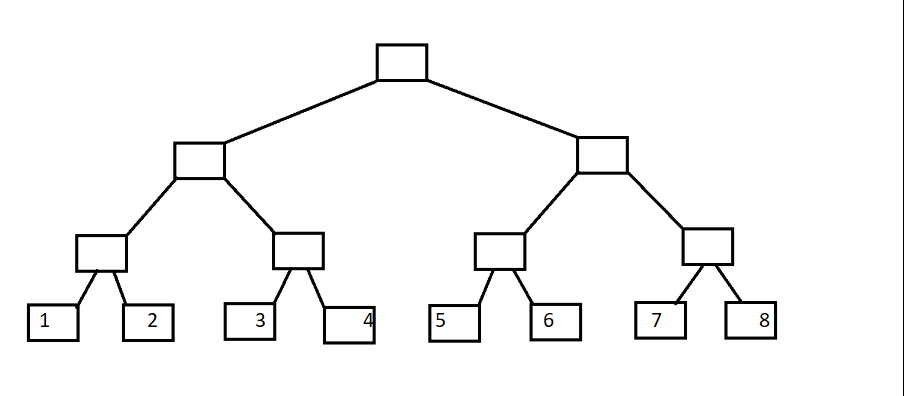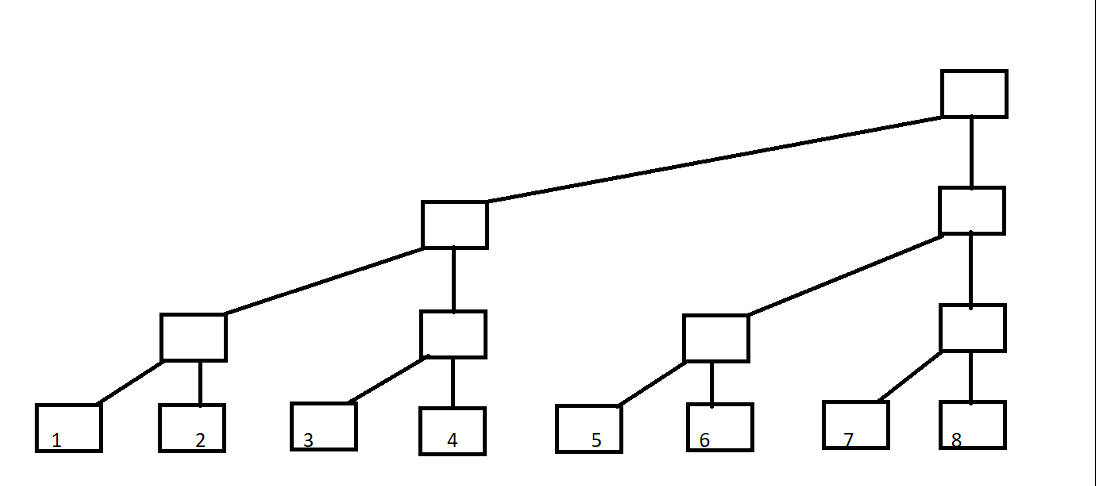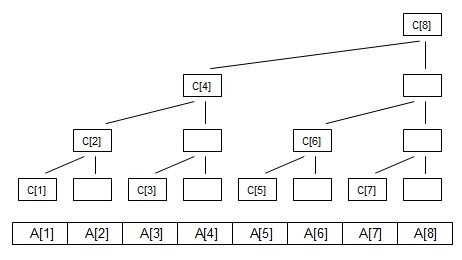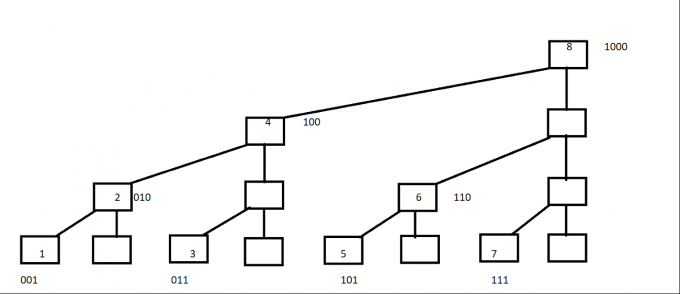# 题解

## 树状数组C[i]代表子树的叶子结点的权值之和

C=A;
C=A+A;
C=A;
C=A+A+A+A;
C=A;
C=A+A;
C=A;
C=A+A+A+A+A+A+A+A;1=(001)---C=A;
2=(010)---C=A+A;
3=(011)---C=A;
4=(100)---C=A+A+A+A;
5=(101)---C=A;
6=(110)---C=A+A;
7=(111)---C=A;
8=(1000)---C=A+A+A+A+A+A+A+A;